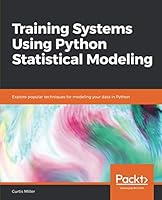# Training Systems Using Python Statistical Modeling0 Reviews
2019-05-20
290 pages

## Book Description

Leverage the power of Python and statistical techniques for building accurate predictive models

#### Key Features

• Get introduced to Python's rich suite of libraries for statistical modeling
• Implement regression, clustering and train neural from scratch
• Includes real-world examples on training end-to-end machine learning in Python

#### Book Description

Python's ease of use and multi-purpose nature has led it to become the choice of tool for many data scientists and machine learning developers today. Its rich libraries are widely used for data analysis, and more importantly, for building state-of-the-art predictive models. This book takes you through an exciting journey, of using these libraries to implement effective statistical models for .

You'll start by diving into classical statistical analysis, where you will learn to compute descriptive statistics using pandas. You will look at supervised learning, where you will explore the principles of machine learning and train different machine learning models from scratch. You will also work with binary prediction models, such as data classification using k-nearest neighbors, decision trees, and random forests. This book also covers for regression analysis, such as ridge and lasso regression, and their implementation in Python. You will also learn how neural networks can be trained and deployed for more accurate predictions, and which Python libraries can be used to implement them.

By the end of this book, you will have all the knowledge you need to design, build, and deploy enterprise-grade statistical models for machine learning using Python and its rich ecosystem of libraries for predictive analytics.

#### What you will learn

• Understand the importance of statistical modeling
• Learn about the various Python packages for statistical analysis
• Implement algorithms such as Naive Bayes, random forests, and more
• Build predictive models from scratch using Python's scikit-learn library
• Implement regression analysis and clustering
• Learn how to train a neural in Python

#### Who this book is for

If you are a data scientist, a statistician or a machine learning developer looking to train and deploy effective machine learning models using popular statistical techniques, then this book is for you. Knowledge of Python programming is required to get the most out of this book.

1. Classical Statistical Analysis
2. Introduction to Supervised Learning
3. Binary Prediction Models
4. Regression Analysis and How to Use It
5. Neural Networks
6. Clustering Techniques
7. Dimensionality Reduction

## Book Details

• Title: Training Systems Using Python Statistical Modeling
• Author:
• Length: 290 pages
• Edition: 1
• Language: English
• Publisher:
• Publication Date: 2019-05-20
• ISBN-10: 1838823735
• ISBN-13: 9781838823733